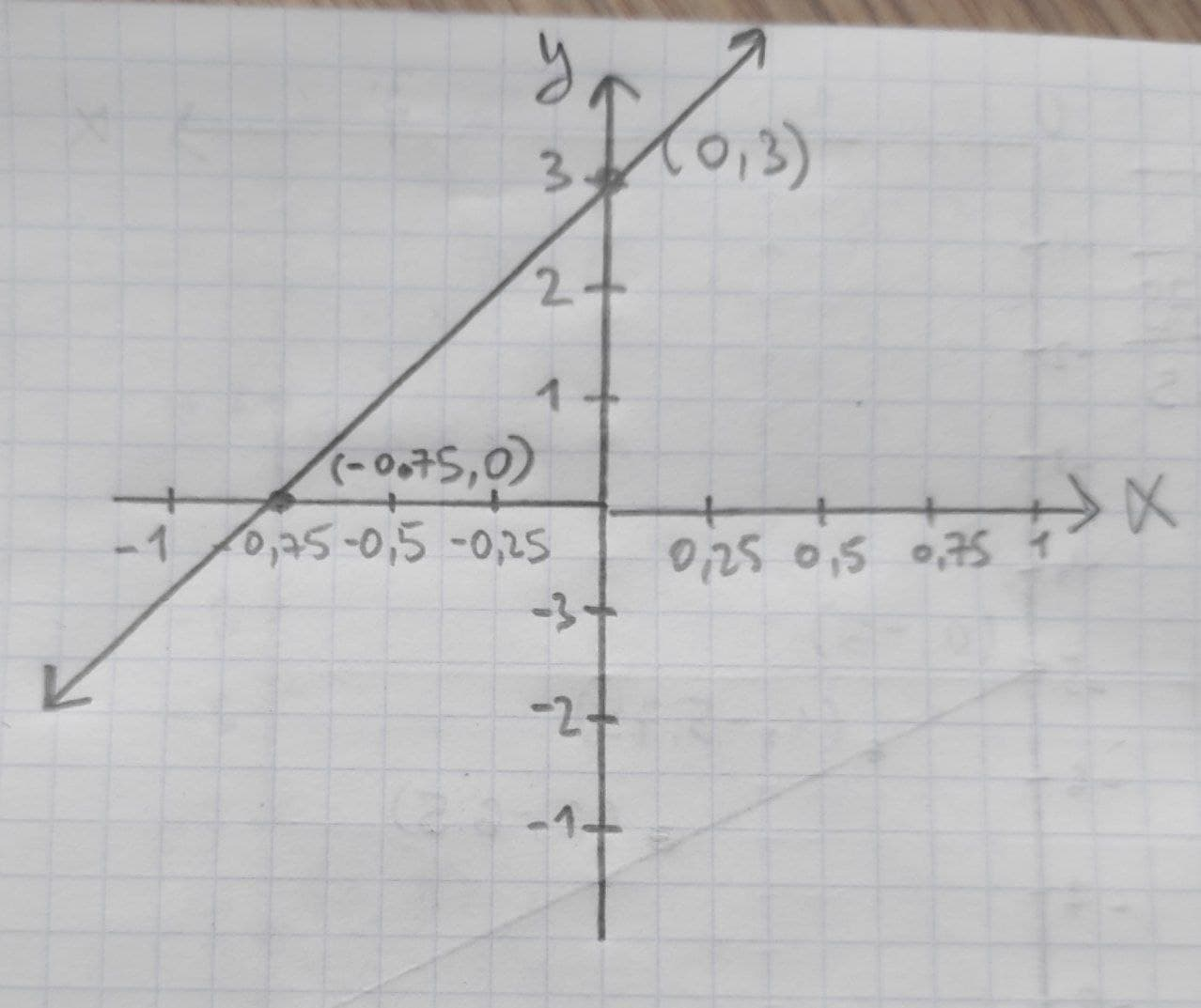# Given linear equation y = 3+4x a. find the y-intercept and slope. b. determine whether the line slopes upward, slopes downward, or is horizontal, without graphing the equation. c. use two points to graph the equation.Tazmin Horton 2020-11-02 Answered
Given linear equation y = 3+4x
a. find the y-intercept and slope.
b. determine whether the line slopes upward, slopes downward, or is horizontal, without graphing the equation.
c. use two points to graph the equation.

• Questions are typically answered in as fast as 30 minutes

### Plainmath recommends

• Get a detailed answer even on the hardest topics.
• Ask an expert for a step-by-step guidance to learn to do it yourself.dessinemoie
The provided linear equation is y = 3 + 4x. The general form of the linear equation is $$\displaystyle{y}={b}_{{0}}+{b}_{{1}}{x}.$$
(a). On comparing the provided linear equation with the general form of the equation, the y-intercept, $$\displaystyle{b}_{{0}}$$ is 3 and the slope, $$\displaystyle{b}_{{0}}$$ is 4.
(b). The slope of the line is +4, so the gradient is +4. The slopes upward as the line moves from left to right.
(c) Considering the provided equation, the values for x=0 and y=0 is obtained as: